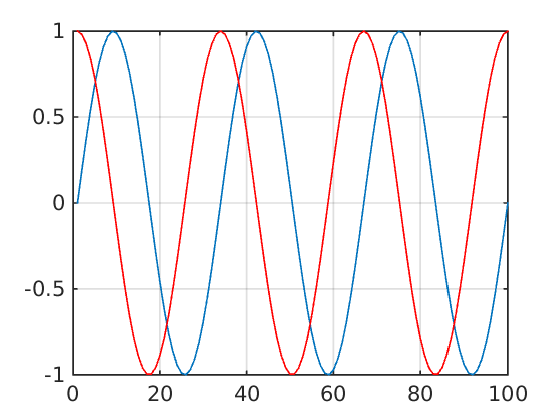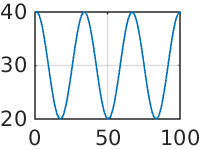# Jupyter Matlab Kernel¶

Interact with Matlab in Notebook the using the Matlab engine for Python. All commands are interpreted by Matlab. Since this is a MetaKernel, a standard set of magics are available. Help on commands is available using the %help magic or using ? with a command.

In :
t = linspace(0,6*pi,100);
plot(sin(t))
grid on
hold on
plot(cos(t), 'r')Out:
In :
%plot -s 200,150

In :
b = 10*cos(t)+30; plot(b); grid onOut:
In :
a = [1,2,3]

Out:
a =

1     2     3
In :
b = a + 3;

Out:
In :
disp(b)

Out:
4     5     6
In :
%lsmagic

Available line magics:
%cd  %connect_info  %download  %edit  %get  %help  %html  %install  %install_magic  %javascript  %kernel  %kx  %latex  %load  %ls  %lsmagic  %magic  %parallel  %plot  %pmap  %px  %python  %reload_magics  %restart  %run  %set  %shell  %spell

Available cell magics:
%%debug  %%file  %%help  %%html  %%javascript  %%kx  %%latex  %%processing  %%px  %%python  %%shell  %%show  %%spell  %%time

In :
%%python
print('Hello, world!')

Hello, world!

In [ ]:


In [ ]: